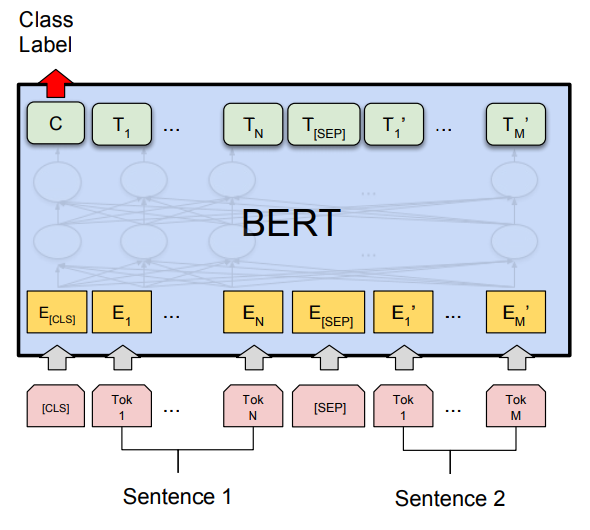B.E.R.T.: The revolutionary language model for NLP

INTRODUCTION-

LSTM networks were the most common deep learning networks which were used for sentiment analysis, next-word prediction. LSTM networks, although good, had a lot of drawbacks, like the text is fed in sequentially and generated sequentially either from right to left or left to right. Even in the case of bidirectional LSTM, the text is learned sequentially from right to left and left to right. The problem is that the true context may be lost during this. Apart from context loss, these networks are also slower to train as they process input sequentially. Transformers were introduced to address some of these concerns, like loss of context to some extent and slower training. Transformers processes the input sentence in one go and not sequentially as in LSTM, so it trains faster and preserves the context. A transformer contains two separate parts, encoder and decoder networks. A series of encoder networks combined sequentially is called B.E.R.T. (Bidirectional Encoder Representation from Transformers).

B.E.R.T. can be used to solve NLP problems such as Neural machine translation, question answering, sentiment analysis, text summarization, etc. Since B.E.R.T. reads the entire sentence at once, it has a deeper understanding of the sentence. Therefore, it is considered bidirectional; this training type allows the model to learn context from all the surrounding words.

ARCHITECTURE AND WORKING-

B.E.R.T. model is trained in two different steps: pretraining and fine-tuning.PRETRAINING

This step involves B.E.R.T. training in two phases- Masked Language Modelling(MLM) and Next Sentence Prediction(NSP).

In Masked Language Modelling, the model takes an input sentence with some words masked( say 10%), and it then tries to predict those words. The masked words in a sentence are chosen randomly; this helps the model to understand the bidirectional context better. The T labels are the word vectors for the output sentence.

In Next Sentence Prediction, the model takes in two sentences and tries to determine the order of those sentences (if sentence A comes before B or vice versa). The C label in the architecture diagram is for the next sentence prediction: 1 if sentence B follows sentence A.

FINE-TUNING

Fine-tuning is basically using B.E.R.T. for the specific problem intended to be solved. After pretraining, it gets a sense of language, and this phase leverages the sense of language to solve specific problems by fine-tuning.

For example, in the classification case, adding a fully-connected layer on top of the output layer is done.

In the case of question-answering tasks, we modify the input and pass the question followed by the paragraph which contains the answer, and the output contains the start and the end word of the sentence that contains the answer.

CONCLUSION

B.E.R.T. has numerous advantages over other deep learning architectures and is proven to perform better in most cases. Still, in some tasks, it might not be feasible to use B.E.R.T. as it is a computationally intensive task and is very costly when the data is large. Despite this, B.E.R.T. is very popular and is being widely used in search engines, etc.

•  February, 10, 2021